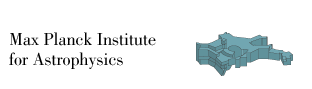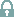# Neutrino emission characteristics of black hole formation in three-dimensional simulations of stellar collapse

Walk, L., Tamborra, I., Janka, H.-T., Summa, A. (2019), arXiv eprint 1910.12971.

## Movies

### Time evolution of the relative electron antineutrino luminosity

Time evolution of the electron antineutrino luminosity normalized to its average [(Lν̅e - <Lν̅e>)/<Lν̅e>] on a Mollweide map of the progenitor's emission surface for the 40 Msun and 75 Msun BH-forming models.

## Neutrino data

### Angle dependent neutrino emission properties

Luminosity (erg/s), mean energy (MeV), and mean squared energies (in MeV2) of νe, ν̅e, and νx for the 40 Msun and 75 Msun BH-forming models. The neutrino properties have been projected and recorded as seen by a distant observer, and mapped to a spherical grid consisting of angular zones in θ (inclination angle) and ϕ (azimuthal angle). The properties have been extracted from the simulation grid at a radius of 500 km.

Each files contain post-bounce time (s), inclination angle (θ), azimuthal angle (ϕ), and the neutrino property at hand, recorded in four columns respectively. At each post-bounce time, the neutrino properties are recorded across all angular zones, ordered firstly by increasing θ values, and secondly across all ϕ coordinates.Download neutrino data for the 40 Msun modelDownload neutrino data for the 75 Msun model

The following (θ,ϕ) directions of the simulation grid correspond to the ones with strong, intermediate, and weak signal modulation (see Figs. 3-4 of our paper):

- s40: direction 1: (1.553, 0.9817), direction 2: (2.9452, 0.5176), direction 3: (2.0528, -0.33914)
- u75: strong modulations: (2.5946, -1.5568), weak modulations: (1.2202, -0.46282)

### Spherically averaged neutrino emission properties

Spherically averaged luminosities (erg/s), mean energies (in MeV), and mean squared energies (in MeV2) [the energies are measured for the number flux] of the aforementioned modelsDownload neutrino data for the 40 Msun modelDownload neutrino data for the 75 Msun model